# Complete Feature Selection Techniques 4-1 Statistical Test & Analysis

Explain and demonstrate Mutual Information, Chi-Square Test, ANOVA F-Test, Regression t-Test and Variance Check for model feature selection

Complete Feature Selection Techniques

1. Statistical Test & Analysis
2. Correlation Analysis
3. Dimension Reduction
4. Model Driven

# Mutual Information (MI)

In statistics, Mutual Information (MI) of two random variables is a measure of the mutual dependence between the two variables. MI is equal to zero if two random variables are independent, and higher values mean higher dependency.

For feature selection, we can use MI to measure the dependency of a feature variable and target variable. MI can be represented as below:

I(x , y) = H(y) - H(y|x)

H is entropy

The intuition for I(x, y) is, If we use y for target variable and x for a feature variable, then I(x, y) represents how much target uncertainty(entropy) reduced if we know feature x.

I(x,y) is also call information gain if we know x to predict y

The following example use a prepossessed Titanic data to demo how to calculate MI for feature selection.

In order to verify the valid of MI, I add a random feature which suppose to have no relationship to target and its MI value should be 0

`import pandas as pdimport numpy as npimport matplotlib.pyplot as pltimport seaborn as snsfrom sklearn import preprocessingfrom sklearn import feature_selection as fs%matplotlib inlinetraining_df = pd.read_csv('Titanic_Preprocessed.csv')# Change the categorical feature value into numeric valuesex_map = {'male':0, 'female':1}training_df['Sex'] = training_df['Sex'].map(sex_map)embarked_map = {'C':0, 'Q':1, 'S':2}training_df['Embarked'] = training_df['Embarked'].map(embarked_map)deck_map = {'A':1,'B':2,'C':3,'D':4,'E':5,'F':6,'G':7,'T':8,'Unknown':9}training_df['Deck'] = training_df['Deck'].map(deck_map)le = preprocessing.LabelEncoder()training_df['Title'] = le.fit_transform(training_df['Title'])# Add a random feature to verify MItraining_df['Random'] = np.random.randint(1, 5, training_df.shape)training_df.head(10)`
`mi_score = fs.mutual_info_classif(    data,     target,     n_neighbors=10,    random_state=22)sorted_idx = np.argsort(mi_score)mi_scoredf = pd.DataFrame(    mi_score[sorted_idx[::-1]],     index=data.columns[sorted_idx[::-1]],     columns=['mi_score'])plt.barh(    data.columns[sorted_idx],     mi_score[sorted_idx])plt.xlabel("Mutual Information Score")`

As expecting, the Random feature(a manually added feature with random value) MI score is very close to 0, and based on all the features MI score, we can choose the best K features.

# Chi-Square Dependence Test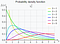Chi-square distribution Wikipedia

In feature selection, we can use Chi-Square independence test to determine if there is dependence between a categorical feature variable and categorical target variable.

Chi-Square Dependence Test is a Hypotheses Test, so we set up below two exclusive assumptions:

1. Null Hypothesis H₀ : Assumes there is no dependence between the two variables, the two variables are totally independent
2. Alternative Hypothesis Hₐ: Assumes there is dependence between the two variables

In Chi-Square Hypotheses testing, we need compare the below results

1. Expected Result - We calculate the result following the H₀ assumption
2. Observed Result - This is what the testing(training) data presents

Let’s use Chi-Square test to check is there any dependency between Sex and Survival in Titanic training data.

First - Create observed contingency table from the training data, the training data is observed data(Titanic data).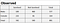Observed Contingency Table

Second - Based on H₀ assumption, sex and survived variable has no dependency relationship, we can calculate expected statistics.

Number of male survive will be (342/891)*577 = 221.47

Number of male not survive will be (549/891)*577=355.53

Same calculation way for female, so we can get expected contingency table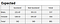Expected Contingency Table

Chi-Square value formula is as below

For our example, Chi-Square value is

X² = (109–221.47)²/221.47 + (468–355.53)²/355.53 + (233–120.53)²/120.53+ (81–193.47)²/193.47=57.12 +35.58 + 104.95 + 65.38= 263.03

Degrees of Freedom(DF) is (2–1) * (2–1) = 1

Third - Check Decision Rule

The DF=1 Chi-Square distribution is as below

Because our example X² = 263.03 > 3.84, which falls in the p=0.05 rejection area, so we reject the H₀ assumption and accept Hₐ assumption, therefore base on the training data, we believe survived has dependency relationship with sex, and we should include sex feature in model.

For p=0.05 rejection, a simple tuition is, base on observe testing results(training data), there is less than a 5% probability the H₀ assumption is correct, so H₀ is rejected.

Python scipy library also provide function to calculate the Chi-Square score and its corresponding p-value

`from scipy import statscontingency_table = pd.crosstab(    training_df.Sex,    training_df.Survived,    margins = False).valueschi2_stat, p_val, dof, ex = stats.chi2_contingency(    contingency_table)chi2_stat, p_val, dof# (260.71702016732104, 1.1973570627755645e-58, 1)`

The output is X² = 260.717 and p-value = 1.197e-58, because p-value < 0.05, so we can reject no independence assumption. (There is small X² difference between 260.717 and my manually calculated result 263.03, this is because rounding in my calculation)

Let’s try to calculate X² for Random feature(a manually added feature with random value) and Survived

`contingency_table = pd.crosstab(    training_df.Random,    training_df.Survived,    margins = False).valueschi2_stat, p_val, dof, ex = stats.chi2_contingency(    contingency_table)chi2_stat, p_val, dof# (3.8388816678946247, 0.2793958179574679, 3)`

The output p-value is 0.279, which is > 0.05, so we can’t reject no independence assumption, therefore we can exclude Random feature in modeling, and this is what we expecting.

# ANOVA F-Test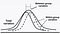https://www.juran.com/blog/analysis-of-variance-anova/

ANOVA means Analysis of Variance, the main purpose of ANOVA is to test if two or more groups differ from each other significantly in one or more characteristics. F-test is another name for ANOVA that only compares the statistical means in groups.

For feature selection, we specifically use One Way ANOVA test, and normally the test is applied on a categorical feature and numeric target.

Intuition of ANOVA F-Test Feature Selection

For example, given a categorical feature “Class” which has three categories A,B,C, and a numeric target “Score

We want to know does feature “Class” has any predictive power for target “Score”?

ANOVA solution is to compare the mean score value in each class category.

Let’s compare the below two extreme Score box-plots for Class category A,B,C

Obviously, if Score distribution for Class category is 2th plot, then the Class feature has very good predictive power, but in 1th plot, it is very hard to predict Score from Class. The conclusion is we need category mean values are well separated apart to have better predictive power.

Based on above intuition, ANOVA F-Test set up the following Hypnosis Assumption:

Null Hypothesis H₀ : All categories mean values are same (i.e μA=μB=μC)

Alternative Hypothesis Hₐ : At least one of the categories mean values differ

If test result can’t reject H₀, then we need drop the feature.

Below is an example using UCI Bike Sharing Dataset Data Set to illustrate F-Test process.

`import numpy as npimport pandas as pdfrom matplotlib import pyplot as pltBike_Sharing_df = pd.read_csv('Bike-Sharing-Dataset\day.csv')Bike_Sharing_df['season'].replace(    {1: 'winter', 2: 'spring', 3: 'summer', 4:'fall'},     inplace= True)# Add a random feature to verify ANOVA TestBike_Sharing_df['random'] = np.random.randint(    1,     5,     Bike_Sharing_df.shape)Bike_Sharing_df[["dteday","season","random","cnt"]].head()`

We will manually calculate F-Test score for feature(x) season and target(y) cnt, based on below F Score formula,

1.Calculate SST - SST is Total Sum of Squares

`grand_mean = Bike_Sharing_df['cnt'].mean()SST = ((Bike_Sharing_df['cnt'] - grand_mean)**2).sum()`

SST = 2739535392.0465117

2. Calculate SSG - SSG is Between Group(or Category) Sum of Squares

`ssg_df = Bike_Sharing_df\    .groupby('season')['cnt']\    .agg({'count','mean'})\    .reset_index()\    .rename(columns={'mean':'category_mean'})ssg_df['ssg'] = (ssg_df['category_mean'] - grand_mean)**2*ssg_df['count']SSG = ssg_df['ssg'].sum()`

SSG = 950595868.4582922

3. Calculate SSE - SSE is Within Groups Sum of Squares

SSE = SST - SSG = 2739535392 - 950595868 = 1788939524

4. Calculate dfG - dfG is degrees of freedom for between groups

`Bike_Sharing_df['season'].value_counts()`

dfG = Number of groups - 1 = 4 - 1 = 3

5. Calculate dfE - dfE is sum of degrees of freedom of all groups, freedom of each group is number of instances in that group minus 1

dfE = (188–1) + (184–1) + (181–1) + (178–1) = 727

6. Calculate F Score

F = (SSG/dfG)/(SSE/dfE) = (950595868/3)/(1788939524/727)

F = 128.7696

7. Calculate Critical Value with P-Value = 0.05 from One Way F Distribution

`import scipy.statscritical_value = scipy.stats.f.ppf(q=1-0.05, dfn=3, dfd=727)critical_value`

Critical Value is 2.617

Because our F score is 128.7696 > 2.617, so we reject Null Hypothesis H₀, therefore we may need to include feature season in modeling.

Let’s calculate F Score for feature random(the feature we add with random category), and this time we will use Python library to calculate F-Score.

`import scipy.stats as statsstats.f_oneway(    Bike_Sharing_df['cnt'][Bike_Sharing_df['random'] == 1],    Bike_Sharing_df['cnt'][Bike_Sharing_df['random'] == 2],    Bike_Sharing_df['cnt'][Bike_Sharing_df['random'] == 3],    Bike_Sharing_df['cnt'][Bike_Sharing_df['random'] == 4])`

The output for random feature

`F_onewayResult(statistic=1.7111487734775328, pvalue=0.1632934027349846)`

F Score is 1.71, and P-value is 0.16

Because P-value 0.16 > 0.05, so can’t reject Null Hypothesis H₀, therefore we need exclude random feature as model feature, and this is what we expecting.

# Linear Regression T-test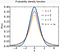t-distribution Wikipedia

Let’s see a linear regression example for Boston House

`import numpy as npimport pandas as pdimport statsmodels.api as smfrom sklearn.datasets import load_bostonboston = load_boston()X = pd.DataFrame(boston.data, columns=boston.feature_names)y = boston.targetx_features = sm.add_constant(X)ols_model = sm.OLS(y, x_features)fit_results = ols_model.fit()print(fit_results.summary())`

Below is the Regression Results

Some information for the above regression results

1. Feature coefficient P value is calculated from t-statistic in t-distribution.
2. The t-test Null Hypothesis H₀ is Coefficients = 0

In the result table, INDUS and AGE features both have P value larger than 0.05, so in t-Test, we can’t reject H₀ Hypothesis(Coefficients = 0), therefor we need remove the two features from regression model.

Also for INDUS and AGE, both coefficient ranges(with 95% confidence level) include 0, coefficient is 0 means there is no relationship and should not include in model.

In summary, in regression model, we can use coefficient P value to select (<0.05) or drop(>0.05) features.

# Variance Check

Variance check for feature selection is to removes all low-variance features.

Variance is information

If a feature is constant value or has very low variance, then the feature can’t provide any information for model prediction, and we need remove it.

# Summary

This story only give basic intuitions for the usage of statistic test and analysis for feature selection, one important matter I don’t include is the conditions for those statistic test, so be careful that when you use those testing.

# REFERENCE

Data Scientist & Engineer from Sydney

## More from Summer Hu

Data Scientist & Engineer from Sydney